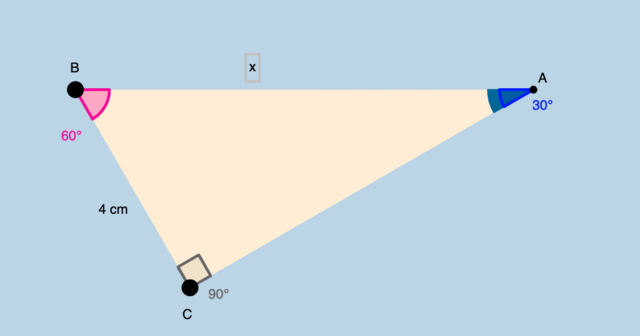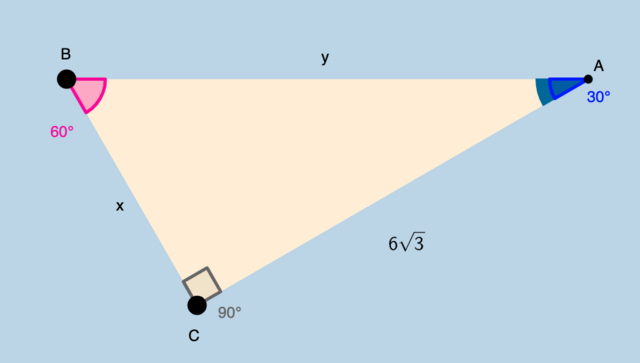# 30 - 60 - 90 Triangles

Take a few minutes to interact with the applet below. Then, answer the questions that follow.

1. How does the length of the hypotenuse of this triangle compare with the length of this triangle's shorter leg?﻿ 2. Suppose the shorter leg's length (BC) = 4 cm. What would AB be? Write this distance in simplest radical form.

Select all that apply
• A
• B
• C
• D
Check my answer (3)

3. Take the information from the previous question. Use this information to find AC. Write this distance in simplest radical form.﻿ 4. Suppose AC = . What is BC? Write in simplest radical form.

Select all that apply
• A
• B
• C
• D
Check my answer (3)

5. Suppose AC = . What is BA? Write in simplest radical form.

Select all that apply
• A
• B
• C
• D
Check my answer (3)﻿ 6. Suppose AC = 5. What is BC? Write in simplest radical form.

Select all that apply
• A
• B
• C
• D
Check my answer (3)